Evaluation Hardware \$300.00## Design Resources

### Design & Integration Files

• Schematic
• Bill of Materials
• Gerber Files
• Allegro layout files
• Assembly Drawing
Download Design Files 648.1 K

### Evaluation Hardware

Part Numbers with "Z" indicate RoHS Compliance. Boards checked are needed to evaluate this circuit

• EVAL-CN0390-EB1Z (\$300.00) 20 GHz to 37.5 GHz, RF Automatic Gain Control AGC Circuit

## Features & Benefits

• 20GHz to 37.5GHz RF Automatic Gain Control
• Wide input amplitude range
• Low phase noise

## Circuit Function & Benefits

The automatic gain control (AGC) circuit is useful in multiple applications such as amplitude stabilization of a synthesizer, controlling output power in a transmitter, or optimizing dynamic range in a receiver. The circuit shown in Figure 1 uses the ADL6010 detector, along with the HMC985A voltage variable attenuator (VVA) and the HMC635 RF amplifier, to provide automatic gain control over a wide range of input frequencies (20 GHz to 37.5 GHz) and amplitude. Circuit performance, as measured by the AGC figures of merit described in this circuit note, are very good between 20 GHz and 30 GHz. The overall gain of the circuit falls off above 30 GHz. However, improvements can be made over narrow bands by using matching techniques not explored in this circuit note.

The AGC circuit has applications in microwave instrumentation and radar-based measurement systems.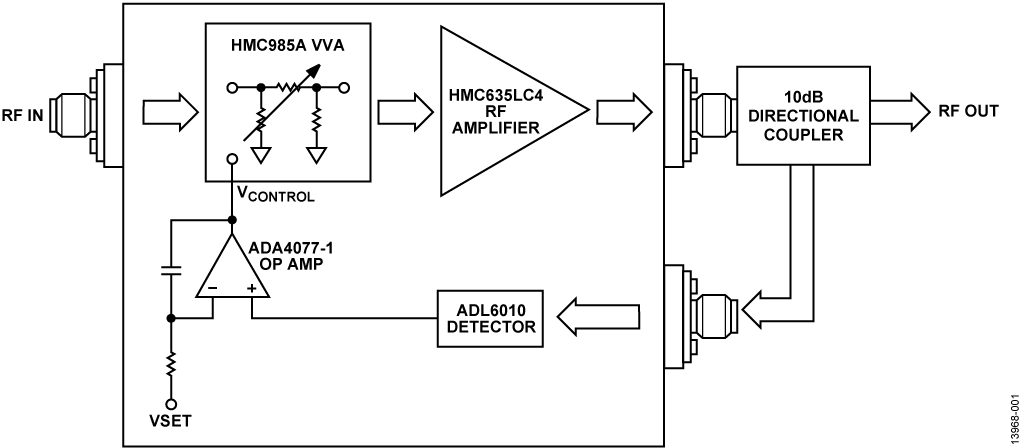Figure 1. Circuit Block DiagramFigure 2. EVAL-CN0390-EB1Z AGC Printed Circuit Board (PCB) Photo

## Circuit Description

### AGC Application Space

Many RF applications require very exact amplitude control with minimal drift over time and temperature. Examples of applications with this requirement are instruments where NBS traceable calibration is required, and where calibration intervals may be long terms such as once or twice per year. Other applications include phased array radar, where the accuracy of the amplitude and phase control limits the beam forming accuracy. The approach used in this circuit, using an op amp in an integrating circuit for the loop controller, provides excellent gain control to compensate for variations in gain of the RF components over input amplitude, RF frequency, and temperature.

In operation, the VSET dc bias controls the output amplitude. The most likely application drives this dc bias with an 8-bit to 12-bit DAC, depending on the accuracy required of the loop. This approach allows digital control of the RF output amplitude. Although the DAC is not included as part of this circuit note, there are many options available, such as the AD5621 12-bit nanoDAC from Analog Devices, Inc.

### Theory of AGC Operation

The central idea behind an AGC circuit of this type is to stabilize the amplitude of an RF signal that may vary based on frequency, temperature, or time. Typically, this circuit has two inputs. The first input is an RF input of a given amplitude whose envelope requires stabilization. The second input is a dc control applied to what is called the VSET input, and it is this input that is used to set the output amplitude. This simple loop is shown in Figure 3.Figure 3. Simple AGC Loop

Figure 3 shows that the difference amplifier is used to compare the VSET voltage to a voltage generated by the detector circuit. The detector converts the RF amplifier output amplitude to a dc voltage. Because the RF input (X) is injected in the middle of the loop, the effect of any variation at X is minimized at the RF output (Y). This effect is true as long as the total loop gain remains high. This effect is explained by the following equations:where Gd is the detector gain.

From Equation 5, it can be seen that the amplitude of X has a minimal effect on the amplitude of Y as long as the value Gd/10 >> 1. Two things that can affect this relationship between X and Y are the gain of the detector, Gd, as well as the 10 dB tap on the directional coupler.

In the CN-0390 design, however, because an integrator is built around the op amp controller circuit, the dc gain of the loop is only limited by the high open-loop dc gain of the op amp. This gain is high enough to make the AGC near perfectly flat within the range of the control loop.

As with any AGC circuit, there are limitations on the operation of the loop. The loop closes for a given range of RF input amplitudes and VSET control voltages. These limitations vary with frequency, as well. In general, the loop is closed and the output amplitude remains flat with RF input variation when the VGAINCTRL (Z) node is between −2.4 V and 0 V, which is the input range of the HMC985A VVA.

Note that the transfer functions for both the detector (VOUT vs. RF input amplitude) and the VVA (attenuation vs. voltage control) are very nonlinear (see Figure 5, Figure 6, and Figure 7), and that their combined gain can vary significantly over the input range of the RF input and the VSET input, possibly over frequency and temperature as well. Using the high gain of the integrator in the control loop compensates for these effects.

The circuit, as built, is more complex than can be described by the simple model of Figure 3. In the actual circuit, the VVA function is broken into two components. The first component is an HMC985A VVA, which provides attenuation from approximately 3 dB at VCTRL = −2.4 V to approximately 40 dB attenuation at VCTRL = 0 V. An HMC635 RF amplifier is used for the gain stage, providing 18 dB of gain over the frequency range of interest.

### Intuitive Approach to AGC Operation

Another approach to conceptualizing the response of the AGC loop is to understand that when the loop is closed (−2.4 V > VGAINCTRL > −0 V), the VSET control voltage is equal to the output voltage of the ADL6010 detector. Under this condition, the op amp integrator is balanced, and the integrator capacitor charge is constant, not charging or discharging. If the RF input amplitude changes while VSET is static, the loop responds, charging or discharging the integrating capacitor until equilibrium is reached again.

With the loop at equilibrium, the ADL6010 output is equal to the VSET voltage. The VSET voltage can then be referenced to the transfer function of the ADL6010 (see Figure 5) to find the ADL6010 RF input power that corresponds to this voltage. From there, add 10 dB to this RF input power number, because of the 10 dB tap on the directional coupler, to find the output power that corresponds to a given VSET voltage. Using this method, a table of output power vs. VSET voltage can be created. The transfer curves of the VVA and ADL6010 are very nonlinear; therefore, this method may be easier than establishing a mathematical description of these transfer curves.

### ADL6010 Envelope Detector

The anchor device in this circuit is the ADL6010 envelope detector. The ADL6010 operates over the frequency range of 500 MHz to 45 GHz. As shown in the functional block diagram in Figure 4, the ADL6010 is a diode-based detector. The response curve for the ADL6010, given in Figure 5, is very nonlinear, which makes straightforward analysis of the feedback loop in this circuit difficult.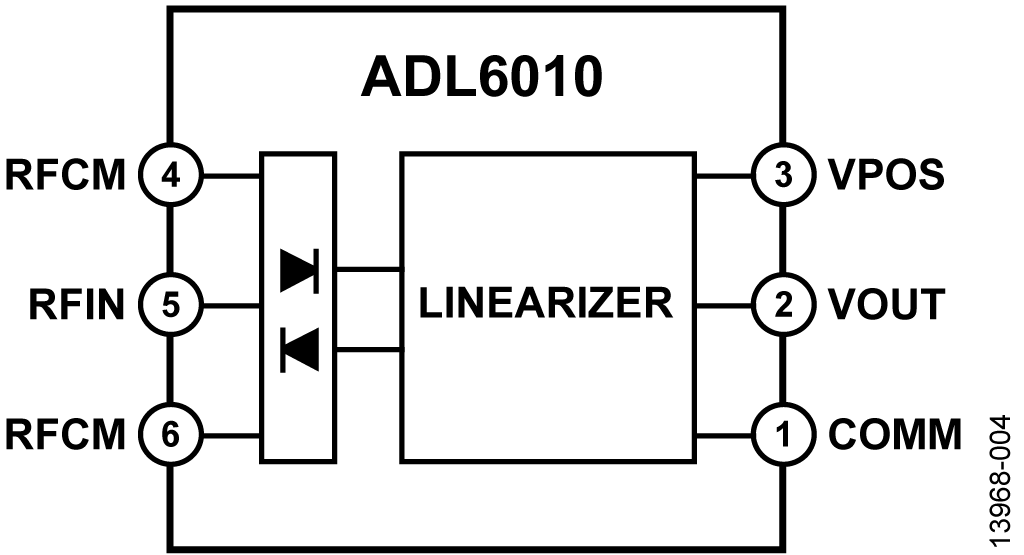Figure 4. ADL6010 Functional Block DiagramFigure 5. ADL6010 Transfer Curve

### VVA and RF Amplifier Combination

The HMC985A VVA operates over the frequency range of 10 GHz to 40 GHz and provides attenuation in the range of 3 dB to near 40 dB. The HMC985A consists of two pi-pad attenuators in series, the first controlled by VCTRL1, the second by VCTRL2. By connecting VCTRL1 and VCTRL2 and driving them together, a combined attenuation of approximately 3 dB to 40 dB is achievable. With the HMC635 RF amplifier in series, the complete voltage controlled gain range is from 15 dB gain to 22 dB of attenuation. The HMC635 is a GaAs amplifier that requires a negative gate voltage (VGG) that must be applied at the same time or before the 5 V VDD supply. Damage can occur to the HMC635 if this VGG requirement is violated. VGG is typically in the range of −0.6 V but may vary slightly from device to device to optimize performance of the amplifier. Consult the HMC635 or other GaAs amplifier data sheets on setting the optimal drain current by adjusting VGG. The evaluation board as built uses a diode and resistor to bias VGG to approximately −0.6 V, for ease of use for the user, so that a separate VGG supply is not necessary. It is recommended that the −5 V supply rail be applied first so that the VGG requirement is met. The user can remove this diode and apply a separate VGG supply to TP6. Moving VGG in a positive direction increases the overall gain of the circuit, but potentially at the cost of added distortion.Figure 6. HMC985A Gain Control Attenuation Curve at 20 GHz, Varying VCTRL1, VCTRL2 = −3 VFigure 7. HMC985A Gain Control Attenuation Curve at 30 GHz, Varying VCTRL2, VCTRL1 = 0 V

### Integrating Op Amp and Details of VSET Control

When the circuit gain is in balance, the ADL6010 detector output voltage equals the externally applied VSET. Figure 8 shows one intuitive method to determine the correct gain sign of the op amp circuit. Label 1 implies that there has been a perturbation in the loop causing the RF output amplitude to increase, most likely an increase in input amplitude. The RF amplitude at the ADL6010 input (Label 2) also increases, as does the voltage at the ADL6010 output (Label 3). The integrator output voltage rises until VSET equals the voltage at the ADL6010 output. The input voltage to the HMC985A VVA (Label 4) therefore increases (on a scale of −2.4 V to 0 V), which in turn increases the attenuation of the VVA, offsetting the positive perturbation at the RF input and verifying that the sign of the feedback control is correct.

The response curve of the ADL6010 can be used to analyze the circuit control range. Because of the op amp integrator function, the loop is in balance when VSET equals the output voltage of the ADL6010. A VSET voltage of 1 V corresponds roughly to 4 dBm RF power into the ADL6010. With the 10 dB tap on the directional coupler, a VSET of 1 V now indicates a power output on the RF amplifier of 14 dBm. By looking at the VVA attenuation curves and taking into account the 18 dB gain of the RF amplifier and understanding that the loop is closed when the VVA voltage is between −2.4 V and 0 V, a table of ideal values of VSET vs. RF output power can be created. Table 1 shows values of expected RF output power vs. VSET, and also shows the expected RF input power range over which the AGC loop is closed. As an example, with VSET = 0.1 V, the loop is at maximum gain (VVA = 3 dB attenuation) for very low signal levels. The knee is reached when the RF input power rises to −20 dBm, at which point the loop closes, and the RF output power remains constant while the RF input power increases. The VVA attenuation limit is reached when the RF input power rises to 17 dBm, at which point the loop opens again, and gain control is lost.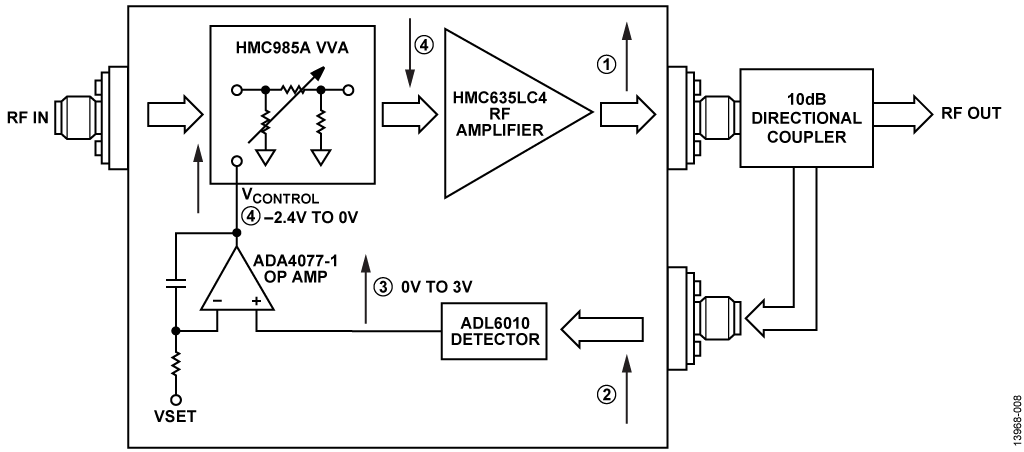Figure 8. Method for Determining Sign of Op Amp Gain Stage

 VSET (V) ADL6010 Input Power (dBm) Add 10 dB for Directional Coupler (dBm) Subtract 21 dB for RF Amplifier Gain and Attenuator Gain1 (dBm) RF In with VATTEN = 0 V (40 dB Attenuation) (dBm) VVA Attenuation Needed for RF Amp input Saturation (dB) 0.1 -15 -5 -20 17 Out of range 0.2 -10 0 -15 Above rated max input power Out of range 0.3 -7 3 -12 Above rated max input power 36 0.4 -5 5 -10 Above rated max input power 34 0.5 -2.5 7.5 -7.5 Above rated max input power 31.5 0.6 0 10 -5 Above rated max input power 29 0.7 1 11 -4 Above rated max input power 28 0.8 2 12 -3 Above rated max input power 27 0.9 3 13 -2 Above rated max input power 26 1.0 4 14 -1 Above rated max input power 25 1 Assuming maximum gain, minimum attenuation of 3 dB.

At the other end of the scale, with VSET = 1.0 V, the knee does not occur until the RF input power reaches 4 dBm, and the loop remains closed while the RF input power reaches the rated maximum input power for the circuit. Note that the data sheet for the HMC635 specifies the P1 dB at 21 dBm; the HMC985A data sheet has characterization plots with up to 24 dBm of input power, and the generator used for this testing has a maximum output power of 20 dBm. Figure 9 shows the ideal plot of VSET vs. expected input and output power and compares it with measured data. Although the ideal does differ slightly from the actual measured data, assuming that the gain of the attenuator and the amplifier are 1 dB to 2 dB less than specified in the data sheet (possibly because of less than perfect RF matching on the this PCB), the ideal matches the measured data very closely. Also, the ADL6010 transfer curve for shown in Figure 5 is for very low frequencies. The ADL6010 compresses slightly at higher frequencies, which accounts for the difference seen between the ideal and measured data at the high end of the VSET scale.

Figure 10 and Figure 11 show the response curves for the AFC circuit at 30 GHz and at 37.5 GHz, respectively. Note that the initial gain drops off at 30 GHz, not much more at 37.5 GHz, and that there is compression of the responses at the higher VSET voltages, limiting the control range of VSET vs. RF output power at these frequencies. At 30 GHz, the plots for the VSET = 0.6 V to VSET =1.0 V overlap each other; at 40 GHz, the plots for the VSET = 0.9 V to VSET =1.0 V overlap each other.Figure 9. ADL6010 AGC Loop Ideal Performance and Measured Performance, at 20 GHzFigure 10. ADL6010 AGC Loop Measured Performance at 30 GHzFigure 11. ADL6010 AGC Loop Measured Performance at 37.5 GHz

### AGC Figures of Merit

There are a number of methods for looking at the quality of an AGC loop.

#### Output Amplitude Flatness over Input Amplitude Variation

Output amplitude flatness over input amplitude variation is the first and most obvious, and the reason for the existence of such a circuit. At low RF frequencies, where the combined gain of the attenuator, RF amplifier, and detector are high enough, virtually perfect flatness is achieved, as shown in Figure 9 and Figure 10. At 37.5 GHz, when the gain of the circuit starts to decay, the flatness is degraded, as shown in Figure 11.

#### Positive Gain

Ideally, an AGC circuit can hold gain flatness for a wide range of output amplitude levels, even with a very small input signal. In reality, there are regions of performance where the overall gain is positive and where the gain is negative. At 20 GHz, as shown in Figure 9, there is a large region where the overall gain is positive. This positive region becomes smaller when the frequency is increased to 30 GHz and then to 37.5 GHz.

#### VSET Range and Linearity

Because of the nonlinearity of the ADL6010 response, the curve of VSET vs. RF output amplitude is also very nonlinear. This nonlinearity is seen at 20 GHz where the curves for the higher VSET voltage are closer together than at lower VSET voltages. At 30 GHz, the VSET vs. output amplitude is compressed enough that the overall AGC response does not change at all above VSET = 0.6 V. The VSET response curve at 37.5 GHz is slightly more open, but is still compressed above VSET = 0.9 V. Figure 12 shows a slightly different way of examining this relationship where the RF output amplitude is plotted vs. VSET at 20 GHz, 30 GHz, and 37.5 GHz.

#### Loop Response to a Transient in Input Amplitude

Stability is a concern for any feedback loop, and an AGC is no different. To obtain an estimate of the stability of this AGC loop, a step was applied to the VSET terminal, and the response was measured at the output of the op amp integrator. As Figure 13 shows, the response is slightly underdamped but indicates good stability. This transient was done with an RF frequency of 20 GHz.

#### Phase Noise

Phase noise can be a problem with analog controlled VGAs, and there is often a trade-off of control voltage input bandwidth and degradation in phase noise. The first step in measuring phase noise degradation is always to measure the generator itself, which is shown in Figure 14 for an input frequency of 20 GHz and amplitude of −10 dBm. A relatively low RF level was chosen so that the loop places itself in a high gain state and maximizes the effects of any phase noise degradation. Figure 15 shows the phase noise at the AGC RF output with a control voltage of 0.1 V, and Figure 16 shows the phase noise at the AGC RF output with a control voltage of 1.0 V. As the results show, there is an insignificant increase in phase noise under these conditions.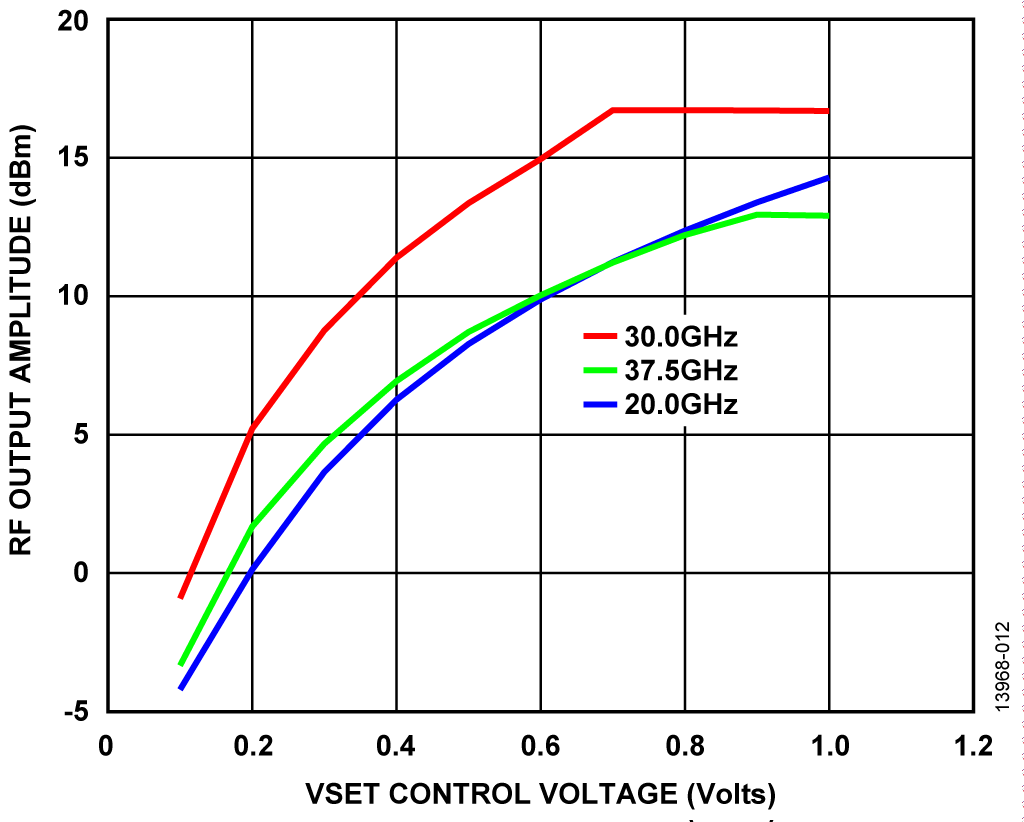Figure 12. RF Output Amplitude vs. VSET vs. Frequency for 19 dBm Input Power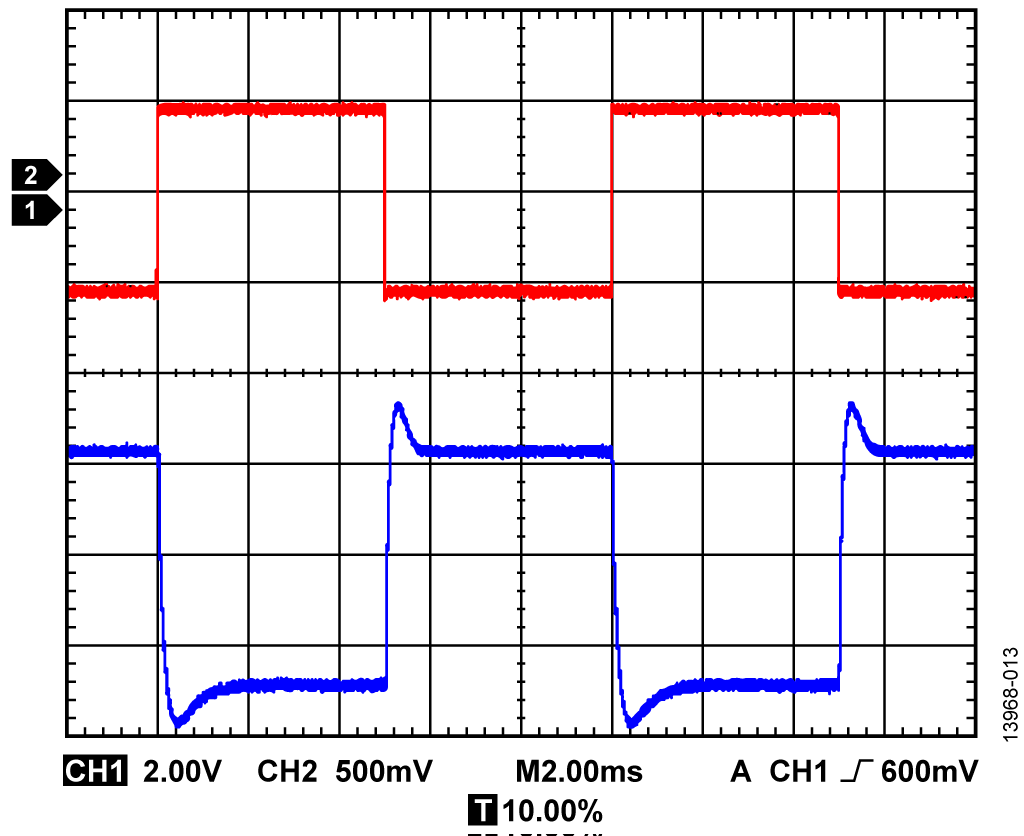Figure 13. Transient Response with Step Applied to VSETFigure 14. Phase Noise Measurement of Generator at 20 GHz, RF Input Power = −10 dBmFigure 15. Phase Noise Measured at AGC RF Output, RF Input = −10 dBm, VSET = 0.1 V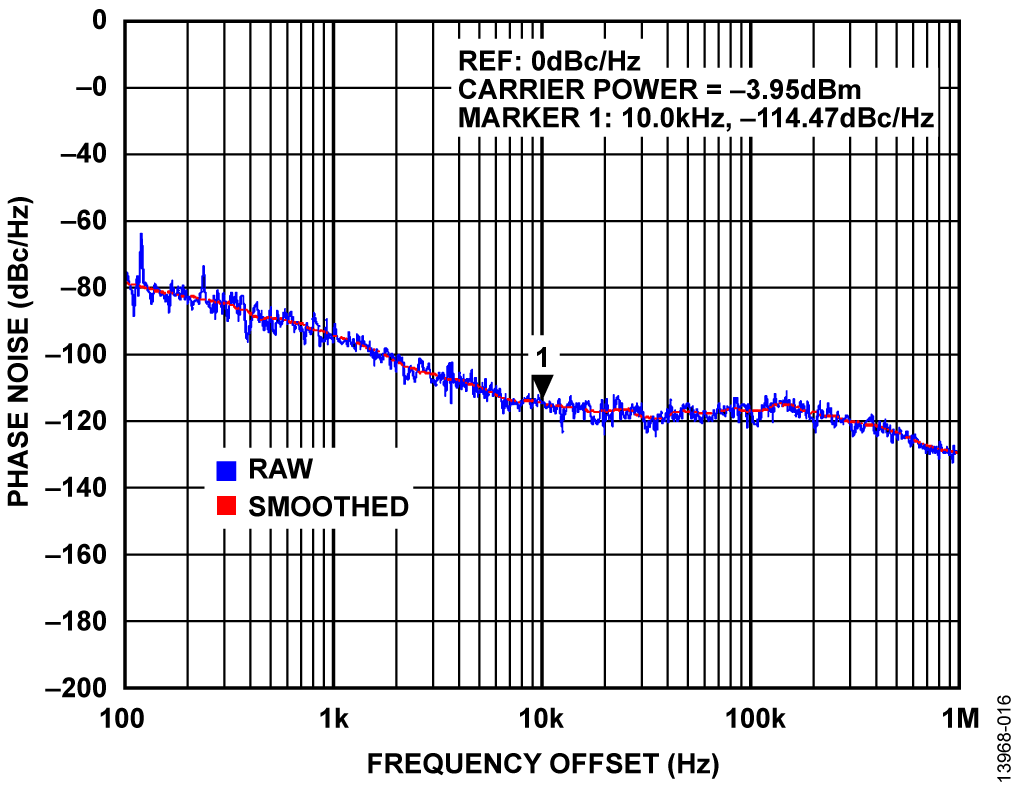Figure 16. Phase Noise Measured at AGC RF Output, RF Input = −10 dBm, VSET = 1.0 V

## Common Variations

This circuit can be built with a number of variations.

The HMC985A VVA and HMC635 RF amplifier were chosen for their wide band capability so that this circuit can be evaluated from 20 GHz to 37.5 GHz. Together, these components provide a gain range of −22 dB to +15 dB with a control voltage of −2.4 V to 0 V. Other combinations of VVA and RF amplifier work over different bands and different gain ranges. The only restriction, because of the power supply range of the loop controlling op amp, is that the VVA control voltage must be in the range of the output voltage of whichever op amp is used for the integrator.

The ADA4077-1 was chosen for low noise (6.9 nV/√Hz) and for its ability to operate from ±5 V supplies. The only absolute requirements for op amps in this application are that they must be a voltage feedback architecture, capable of running from ±5 V, and with an output range capable of driving the desired VVA range.

The gain scaling response can be adjusted by using attenuators on the 10 dB tap of the directional coupler, or a different tap value for the directional coupler. In this situation, the output power is a function of VSET referred to the ADL6010 transfer curve, plus the values of the attenuators and directional coupler tap.

A power splitter can be used rather than a directional coupler. Using a power splitter has the effect of increasing the amplitude at the ADL6010 input, and therefore shifts the response curves shown in Figure 9, Figure 10, and Figure 11. This shift is at the expense of 6 dB of output power.

This board and circuit were designed to cover the band from 20 GHz to 37.5 GHz. Performance over a narrow band can be improved by using matching techniques. Refer to Figure 20 to see where narrowband matching can give the most improvement.

## Circuit Evaluation & Test

All RF cables used in this test must be premeasured for loss up to 40 GHz. The SMA connectors used on the AGC PCB are 2.4 mm; therefore, cables and adapters must be available to mate with these connectors.

### Equipment Needed

The following equipment is needed:

• EVAL-CN0390-EB1Z circuit evaluation board.
• +5 V power supply, 500 mA capability (for ADA4077-1 op amp, HMC635 RF amplifier, and ADL6010 detector).
• −5 V power supply, 100 mA capability (for ADA4077-1 op amp).
• −0.6 V supply for VGG bias on HMC635, 10 mA range required. This supply is optional; a diode and resistor can be used to provide this bias. There are pads for a diode and resistor on the PCB. It is important that this VGG bias be applied before the +5 V bias on the HMC635. The +5 V VDD and the VGG bias must not be applied simultaneously.
• Power supply, adjustable 0 V to 3.0 V for VSET control. Current only necessary in the mA range.
• CN-0390 Evaluation Software (download from ftp://ftp.analog.com/pub/cftl/cn0390/).This software is optional, because the circuit can run completely manually. A C# .exe file and source code are available from Analog Devices. The code is in Microsoft Visual Studio C#, 2012. The code uses GPIB libraries to control the RF generator, the VSET control, and the spectrum analyzer. The GPIB libraries used are from National Instruments, available at no cost. The GPIB (SCPI codes) in the software are specifically for the instruments described in this section; although SCPI codes between similar instruments (different spectrum analyzers, for instance) are often identical, this code may not work if different instruments are used.
• 40 GHz capable continuous wave (CW) signal generator (Keysight or Rohde & Schwarz are recommended)
• Spectrum analyzer with >40 GHz capability (Keysight or Rohde & Schwarz or similar)
• 10 dB directional coupler (Keysight and KRYTAR are recommended)
• Multimeter capable of measuring the control voltage range at the HMC985A VVA input. The range on the multimeter must be −5 V to +5 V because the control loop may rail to the power supplies when the loop is not closed.
• Coaxial RF cables with SMA connectors, ideally lowest loss possible at 40 GHz. The SMA connectors used on the PCB are 2.4 mm connectors, from Southwest Research. To reduce loss, use SMA barrel connectors rather than cabling to connect the directional coupler to the evaluation board.

### Getting Started

To start evaluating the circuit, take the following steps:

1. Preset the RF generator to a frequency of 20 GHz and −20 dBm output power. Disable the generator. Set the spectrum analyzer to a center frequency of 20 GHz, 1 GHz span, reference level of 20 dBm, RBW = 30 kHz.
2. Connect the multimeter to the HMC985A VVA control input.
3. Set the power supplies to their correct values. Set the VSET to 0 V. Disable all power supplies.
4. Connect the RF output of the AGC to the input port on the directional coupler. If possible, make this connection directly with an SMA barrel connector, becauase even a short cable can degrade the overall performance. The 10 dB tap from the directional coupler must connect through as short as possible a cable to the detector input of the AGC PCB.
5. Connect all other cables and supplies as shown in Figure 18.
6. Turn on the −5 V supply first to bias VGG on the HMC635, then turn on the +5 V supply. Both supplies can come up at once if that is convenient; however, the +5 V supply must not come on before the −5 V supply.
7. Check the +5 V, −5 V, and −0.6 V supplies for their current. They must all read similar to the following:
• +5 V supply, 300 mA
• −5 V supply, 10 mA
• −0.6 V supply, if using external, 1 mA or less
8. Turn on the VSET supply. VSET is varied during the course of the evaluation. The current must never exceed 5 mA.
9. Enable the RF generator.

### Running the Circuit in Manual Mode

The circuit is now fully functional. The RF generator must initially be set to an input amplitude of −20 dBm. Do not exceed +20 dBm because that is close to the input compression limit of the HMC635 RF amplifier. With the RF generator power set to −20 dBm, the VVA control voltage may go to the −5 V rail. With very low amplitude signals, the loop maximizes gain, so the integrator output voltage stays below −2.4 V until the RF amplitude is high enough to close the loop. As the RF power is increased, the user at first sees the output power indicated on the spectrum analyzer increase on a dB/dB basis. However, as the RF input power increases and the knee in the AGC response curve is reached, the loop closes and the VVA control voltage moves to −2.4 V. At this point, the loop is closed with minimum signal input, and therefore the loop is still trying to set itself to maximum gain. As the power increases further, there is no noticeable change in amplitude on the spectrum analyzer, because the loop is compensating for the change in input level. Instead, as the power is increased, the user sees the VVA control voltage increase from −2.4 V towards 0 V. Given the gain of the VVA, the RF amplifier, and the detector, the VVA control voltage does not go all the way to 0 V with input power ≤ +20 dBm, and therefore the AGC slope remains flat.

If all the results are as described previously, the circuit can now be evaluated at different RF amplitudes, frequencies, and VSET values.

### Design of the PCB

Designing a PCB for wideband operation from 20 GHz to 40 GHz is not a trivial process. Grounded coplanar waveguide techniques were used for the PCB and a test trace was added to the PCB to verify the 50 Ω trace construction. The overall layout, with power and signal connections, is given in Figure 17. The PCB, in the actual test setup, is shown in Figure 18. Note the connection of the external 10 dB directional coupler, and that the RF input connects directly to the RF generator to eliminate the need to compensate for cable loss.

A complete design support package including layouts, schematics, and bill or materials can be downloaded from www.analog.com/CN0390-DesignSupport.Figure 17. ADL6010 AGC Circuit—PCB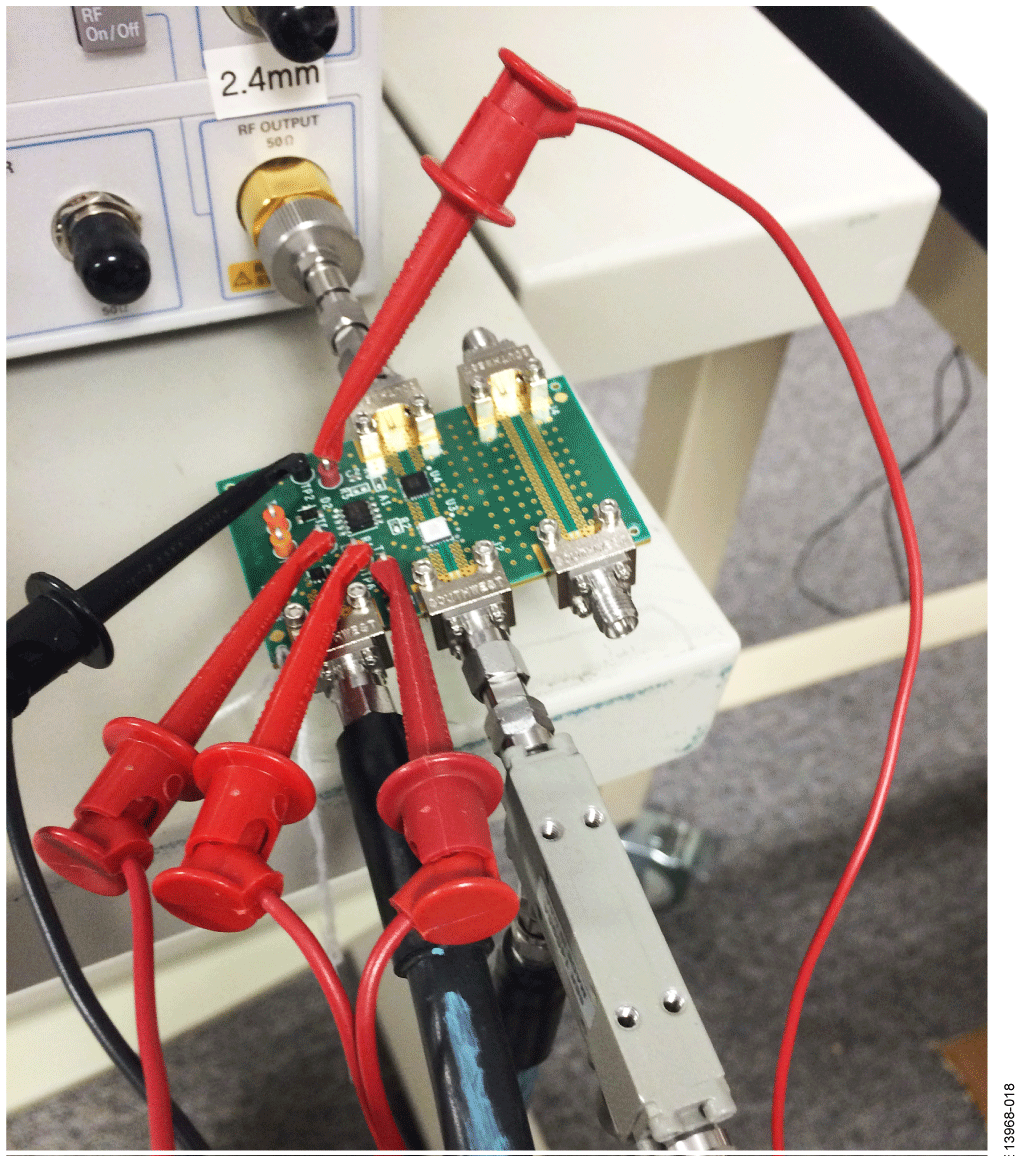Figure 18. ADL6010 AGC Circuit—Test Configuration

### Verification of RF Trace Quality and Circuit S Parameters

The PCB was designed in Cadence Allegro. All CAD design files are available with this circuit note. To achieve 50 Ω trace quality to 40 GHz, the Allegro design files were extracted to and then simulated in ADS.

The S parameters, S11, S22, S21, and S12, are shown in Figure 19. As the data shows, even with the upfront design and simulation, the return loss in the 23 GHz to 28 GHz region is less than optimal. The performance in the 30 GHz to 40 GHz range is much better.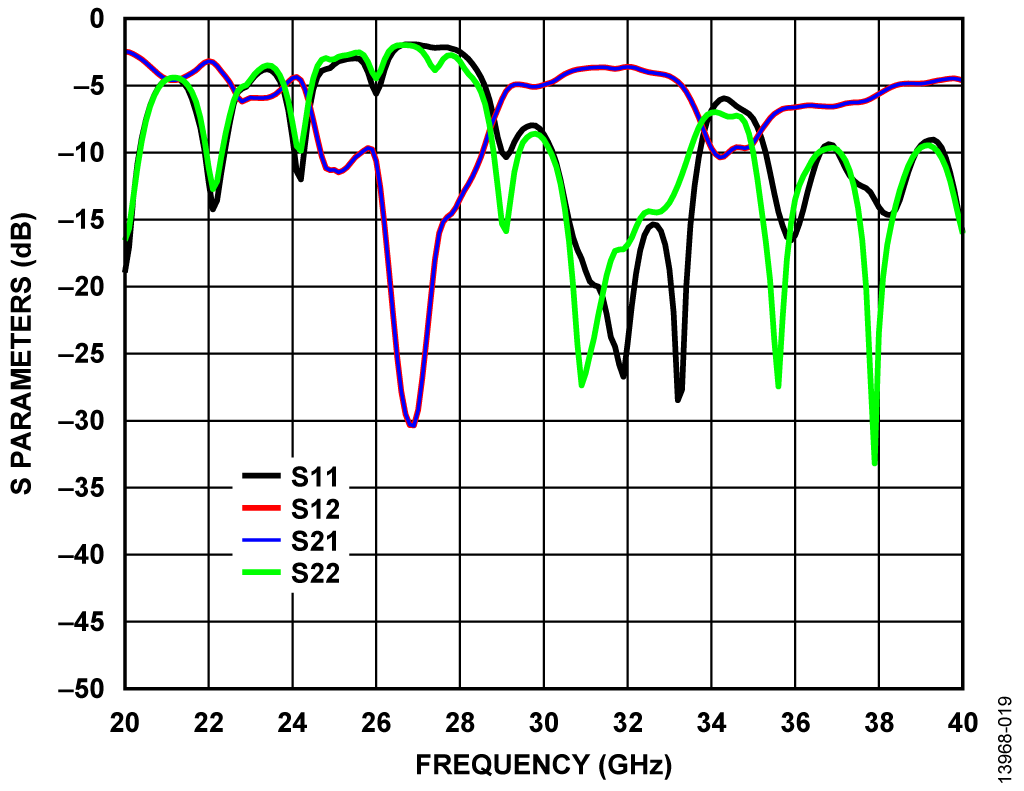Figure 19. AGC PCB—Test Trace S Parameters

Figure 20 shows the two port S parameters (RF in, RF out) for the active circuitry on the PCB. There is roll-off in the gain performance (S21) from 20 GHz to 40 GHz. There are also nulls in the gain performance that align with the return loss issues shown in the test trace.Figure 20. AGC PCB—Active Circuitry S Parameters

## Sample Products

### Samples

 Product Description Available Product Models to Sample ADA4077-1 4 MHz, 7 nV/√Hz, Low Offset and Drift, High Precision Single Amplifier ADA4077-1ARMZ ADA4077-1ARMZ ADA4077-1ARZ ADA4077-1ARZ ADA4077-1BRZ-R7 ADA4077-1BRZ-R7 ADL6010 Fast Responding, 45 dB Range, 0.5 GHz to 43.5 GHz Envelope Detector ADL6010ACPZN-R7 ADL6010ACPZN-R7 ADL6010SCPZN-R7 ADL6010SCPZN-R7
Buy functionality exists in desktop site
Evaluation Boards Pricing displayed is based on 1-piece.
Up to two boards can be purchased through Analog.com. To order more than two, please purchase through one of our listed distributors.
Pricing displayed is based on 1-piece. The USA list pricing shown is for budgetary use only, shown in United States dollars (FOB USA per unit), and is subject to change. International prices may vary due to local duties, taxes, fees and exchange rates.